Courses

# Test: Word Problems

## 10 Questions MCQ Test Mathematics (Maths) Class 11 | Test: Word Problems

Description
This mock test of Test: Word Problems for JEE helps you for every JEE entrance exam. This contains 10 Multiple Choice Questions for JEE Test: Word Problems (mcq) to study with solutions a complete question bank. The solved questions answers in this Test: Word Problems quiz give you a good mix of easy questions and tough questions. JEE students definitely take this Test: Word Problems exercise for a better result in the exam. You can find other Test: Word Problems extra questions, long questions & short questions for JEE on EduRev as well by searching above.
QUESTION: 1

### In drilling world’s deepest hole, it was found that the temperature T in degree Celsius, x km below the surface of Earth, was given by T = 30 + 25 (x – 3), 3 < x < 15. If the required temperature lies between 200o C and 300o C, then the depth, x will lie between

Solution: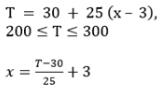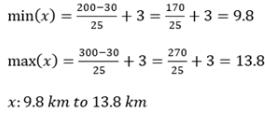QUESTION: 2

### A solution of 10% boric acid is to be diluted by adding a 4% boric acid solution to it. The resulting mixture is to be more than 5% but less than 8% boric acid. If we have 750 litres of the 10% solution, then the quantity of the 4% solution that has to be added will lie between

Solution: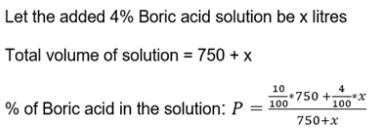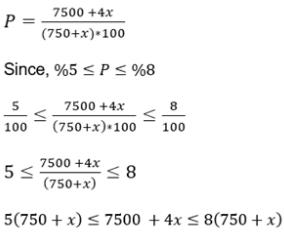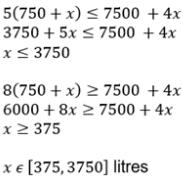QUESTION: 3

### Pairs of consecutive even positive integers, both of which are larger than 7 such that their sum is less than 28, are

Solution:

Let the pair be: (x, x+2)
x + (x + 2) < 28
x < 13
So, Option C satisfies the condition

QUESTION: 4

The longest side of a triangle is 4 times the shortest side and the third side is 4 cm shorter than the longest side. If the perimeter of the triangle is at least 59 cm, then the minimum length of the shortest side is

Solution:
QUESTION: 5

In an experiment a solution of hydrochloric acid is to be kept between 30 and 35 degree celcius. What is the range of temperature in degree Fahrenheit if conversion formula is given by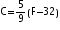,where C and F represent temperature in degree celsius and degree fahrenheit, respectively?

Solution:

Temperature is defined as the degree of hotness.
Temperature is measured using different scales in different regions.
we know that
C = temperature in celcius.
F = temperature in Fahrenheit.
F= 9(C)/5 + 32
1) C = 30
F= 9(30)/5 + 32
F= 54 + 32 = 86F
2) C = 35
F= 9(35)/5 + 32
F= 63 + 32 = 95F
The range of temperatures in degree Fahrenheit is 86 F to 95 F.

QUESTION: 6

A man wants to cut three lengths from a single piece of board of length 91 cm. The second length is to be 6 cm longer than the shortest and the third length is to be thrice as long as the shortest. If the third piece is to be at least 4 cm longer than the second, then the length of the shortest board will lie between

Solution:

Let the length of the shortest piece, The length of the second price is (x+6)cm. The length of the third piece is 3x cm, Total length of the three prieces must be ≤91cm.
xcm+(x+6)cm+3xcm≤91cm
=>5x+6≤91
Subtracting 6 from number 5 on both sides, 5x/5≤85/5
x≤17----(1)
Step 2:
Also third piece is at least 4 cm longer than the second piece. therefore
3x≥(x+6)+4
=>3x≥x+10
Subtracting x from both sides,
3x−x≥10
x≥5 -----(2)
Step 3:
From (1) and (2)
5≤x≤17

QUESTION: 7

A plumber can be paid under two schemes as given below:
I: Rs 600 and Rs 50 per hour.
II: Rs 170 per hour.
If the job takes n hours, then the values of n for which the scheme I will give the plumber better wages are

Solution:

Your question is not complete it should be,
I: &600 fix and &50 per hourNow solving the question,Let the work is done in n hour as per given , So After n hour according to first scheme he will get an amount
= 600+50n After n hour according to second scheme he will get an amount,
=170nNow we are asked for values of n such that scheme
I give better wages so 600+50n >170n600>120nor n <5 .SO if the numbers of hour are less than 5 then he will get better wages by first scheme.

QUESTION: 8

In the first four examinations, each of 100 marks, Dipika got 94, 73, 72, 84 marks. If a final average greater than or equal to 80 and less than 90 is needed to obtain a final grade B in a course, then the range of marks, Dipika must score in the fifth examination in order to obtain grade B is

Solution: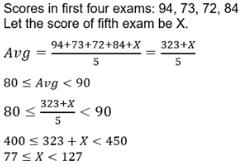QUESTION: 9

The cost and revenue functions of a product are given by C(x) = 2x + 400 and R(x) = 6x + 20 respectively, where x is the number of items produced by the manufacturer. The minimum number of items that the manufacturer must sell to realize some profit is

Solution:

Profit = revenue - cost
But given profit > 0
therefore R(x) - C(x) >0
therefore 4x - 380 >0
therefore 4x > 380
therefore x>95  manufacturer must sell 96 items.

QUESTION: 10

IQ of a person is given by the formula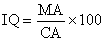, where M A is mental age and CA is chronological age. If 85 < IQ < 145 for a group of 12-year children, then the mental age will lie between

Solution:

85≤MA/CA×100≤145
(85*12)/100≤MA/CA×100≤(145*12)/100
10.2≤MA≤17.4
range of mental age is[10.2,17.4].